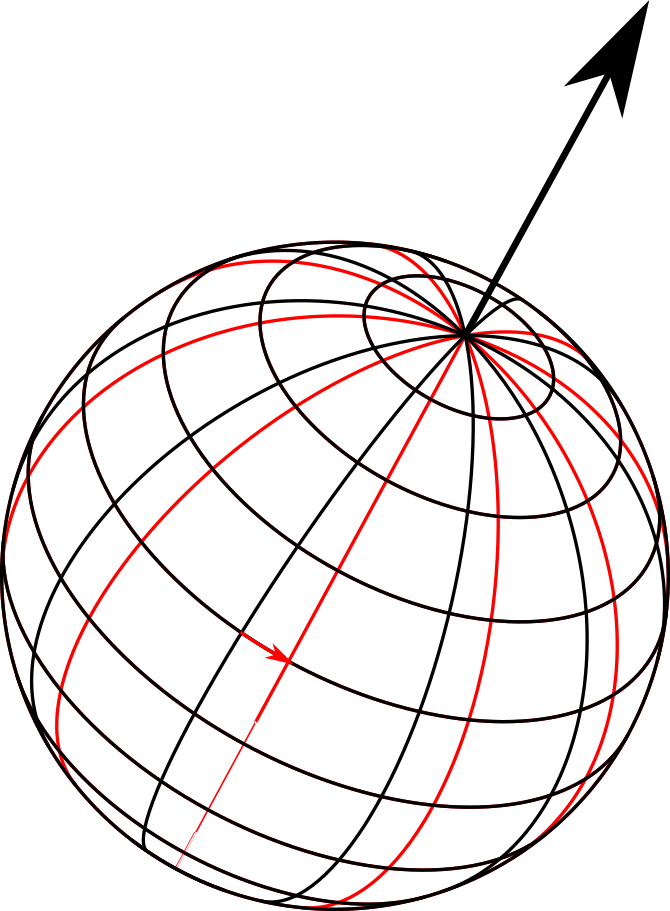# Rotation#

class orix.quaternion.Rotation(data: )[source]#

Bases: `Quaternion`

Transformations of three-dimensional space, leaving the origin in place.

Rotations can be parametrized numerous ways, but in orix are handled as unit quaternions. Rotations can act on vectors, or other rotations, but not scalars. They are often most easily visualised as being a turn of a certain angle about a certain axis.Rotations can also be improper. An improper rotation in orix operates on vectors as a rotation by the unit quaternion, followed by inversion. Hence, a mirroring through the x-y plane can be considered an improper rotation of 180° about the z-axis, illustrated in the figure below.Rotations support the following mathematical operations:
• Unary negation.

• Inversion.

• Multiplication with other rotations and vectors.

Rotations inherit all methods from `Quaternion` although behaviour is different in some cases.

Rotations can be converted to other parametrizations, notably the neo-Euler representations. See `NeoEuler`.

Attributes

 `Rotation.angle` Return the angles of rotation. `Rotation.antipodal` Return this and the antipodally equivalent rotations. `Rotation.axis` Return the axes of rotation. `Rotation.improper` Return `True` for improper rotations and `False` otherwise.

Methods

 `Rotation.angle_with`(other[, degrees]) Return the angles of rotation transforming the rotations to the other rotations. `Rotation.angle_with_outer`(other[, degrees]) Return the angles of rotation transforming the rotations to all the other rotations. `Rotation.dot_outer`(other) Return the outer dot products of the rotations and the other rotations. Return a new rotation instance collapsed into one dimension. `Rotation.from_align_vectors`(other, initial) Return an estimated rotation to optimally align two sets of vectors. `Rotation.from_axes_angles`(axes, angles[, ...]) Initialize from axis-angle pair(s). `Rotation.from_euler`(euler[, direction, degrees]) Initialize from Euler angle set(s) . `Rotation.from_matrix`(matrix) Return rotations from the orientation matrices . `Rotation.from_neo_euler`(neo_euler) Create rotations(s) from a neo-euler (vector) representation. `Rotation.from_scipy_rotation`(rotation) Return rotations(s) from `scipy.spatial.transform.Rotation`. `Rotation.identity`([shape]) Return identity rotations. `Rotation.outer`(other[, lazy, chunk_size, ...]) Return the outer rotation products of the rotations and the other rotations or vectors. `Rotation.random`([shape]) Return random rotations. `Rotation.random_vonmises`([shape, alpha, ...]) Return random rotations with a simplified Von Mises-Fisher distribution. `Rotation.to_euler`([degrees]) Return the rotations as Euler angles in the Bunge convention . Return the rotations as orientation matrices . `Rotation.unique`([return_index, ...]) Return the unique rotations from these rotations.